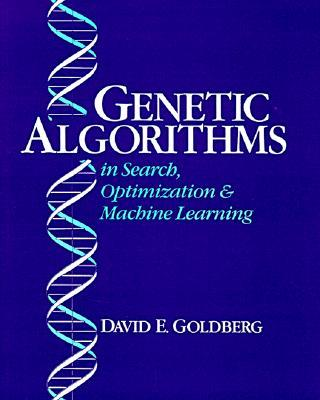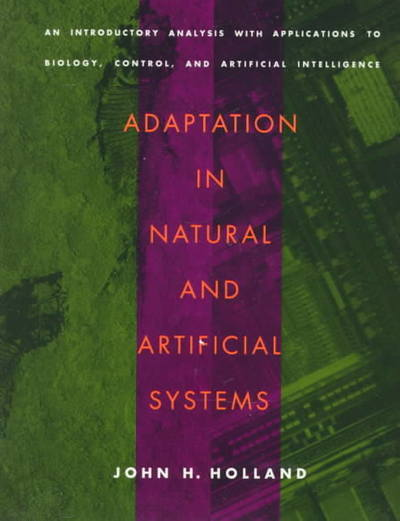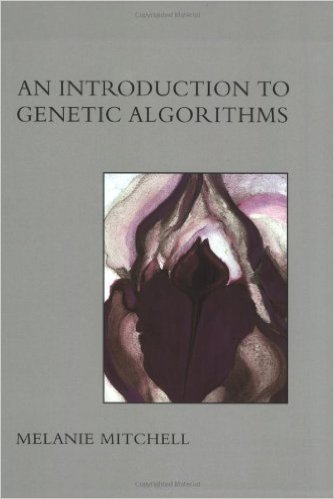Jan van Eijck

# Description

The project will cover part of the material in(Goldberg 1989)

John H. Holland (who died in August 2015) and David E. Goldberg are pioneers in the field of genetic algorithms. The book by Goldberg is from 1989 and has 68350 citations (October 2015), which makes it one of the most cited books in computer science. It is still worth studying, for it gives a very clear introduction to evolutionary programming and its use for search, optimization and machine learning. In the project we will bring the book up-to-date by re-implementing some key genetic search algorithms in the functional programming language Haskell.

# Organisation

The project starts with a brief overview of the principles behind genetic programming, taken from (Goldberg 1989).

Next, there will be a very quick introduction to functional programming, and we will look at available modules for generic programming in Haskell.

The rest of the project will consist of exercise, coding and discussion meetings where we will carry out exercises from (Goldberg 1989), and code up a substantial number of genetic algorithms in different application areas.

The project is closed off with an individual project report.

The students are supposed to use their own laptops for carrying out coding exercises.

# Prerequisites

Programming experience is a pre, but the project can also serve as a crash course in (functional) programming.

# Assessment

In order to pass, the students are required to attend the meetings, carry out the programming assignments, and hand in an individual report about work on the implementation of a genetic algorithm.

The programming assignments and the report will be graded, and the final grade is the average of the report grade and the average for the assigment grades.

# Example Genetic Algorithm

Suppose we have a pile of $$n$$ cards, numbered consecutively from $$1$$ through $$n$$. The task is to divide this pile into two piles $$L$$ and $$R$$, such that ten times the sum of the numbers on the cards in $$L$$ is as close as possible to the product of the numbers on the cards in $$R$$.

The genetic programming approach to this starts with choosing an appropriate representation.

Represent the pile $$L$$ as a list of bits of length $$n$$

 [b,b,b,b, ... , b,b,b]

with each b equal to $$1$$ if the card in the corresponding position is in $$L$$ and equal to $$0$$ otherwise.

A scoring function $$| 10 * \sum L - \prod \overline{L} |$$ calculates the fitness of a bitlist $$L$$. A score of $$0$$ means a perfect fit.

A crossover function combines two parent bitlists into a new bitlist by determining at which position $$k$$ an initial substring from the first parent gets combined with a terminal substring from the second parent.

A mutation function determines when and where a bit is randomly flipped.

The simple genetic algorithm now works as follows:

• Start with an initial population $$P$$.

• While maximum number of generations not reached or score not perfect:

• Determine the fitness of each member of the current population using the score function.

• Select parents from the current population in proportion to their fitness, and use crossover and mutation to generate offspring.

• Replace the old population with the new population.(Holland 1975)(Mitchell 1999)

# Meeting Jan 5

• An implementation of a genetic algorithm solution for the cards program in Haskell is in GAexample.lhs.

• You can run this as main, and you can play with the parameters. In the example, the length of the bitstring is set to 40.

• Exercise: compare this with a solution by means of exhaustive search.

• We agreed to read Chapter 1 of (Goldberg 1989), and to solve the problems on pages 23 and 24, and carry out the implementation exercises on page 25 (but using Haskell instead of Pascal).

• Next meetings: January 7, January 12, January 14. All meetings at 10am.

• The meetings will be devoted to progress reports. Each should be prepared to share his progress with the group.

Goldberg, David E. 1989. Genetic Algorithms in Search, Optimization and Machine Learning. Pearson Education.

Holland, John H. 1975. Adaptation in Natural and Artificial Systems. Ann Arbor: The University of Michigan Press.

Mitchell, Melanie. 1999. An Introduction to Genetic Algorithms. Fifth Edition. MIT Press.